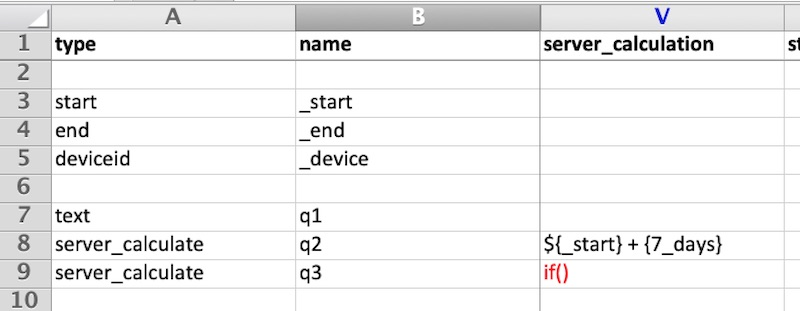# Server Calculations¶

## Introduction¶

Questions can be added to surveys where the answer is calculated on the server and not on the phone. When creating reports or viewing data in the console these calculations appear just like data that was entered in WebForms or on a mobile device. An important difference is that the calculation is performed when the data is viewed. Hence if you change the expression used in the server calculation then the answer shown will change for all previously collected data.

Note

You can also create calculation questions that are performed within a survey by specifying a question ot type "calculate". This is useful if you are going to use the results of that calculation during the data collection process, perhaps in a relevance. However it the calculation is only used during analysis you could consider server calculations. Calculations performed in a survey are fixed once the data is submitted. So if you update the survey to change the calculation the values calculated for already collected data will not change.

Calculations are defined using Server Expressions. The one exception is when using the if() expression which is specific to server calculations.

## Excel Editor¶

In the Survey sheet specify

• type: server_calculate
• name: Specify a unique name name
• server_calculation: Put the calculation here

## If Function¶

One of the most important functions for a server calculate is if(). The excel editor adds additional support to make it easier to specify these. Currently the if() function for server calculates is not supported by the online editor. You also cannot use this if() function in an advanced filter.

In the server_calculate column of the survey sheet enter only: if(). In the following example there are two server calculations the second of which is an if().if() Server Calculations

Then set the conditions and their values in the "conditions" sheet of the excel file.if() Conditions

In the above example the value of q3 will be set to 'A Selected' if the answer to q1 was 'A'; 'B Selected' if the answer to q1 was 'b'; and to 'Something else selected' in all other cases.